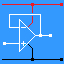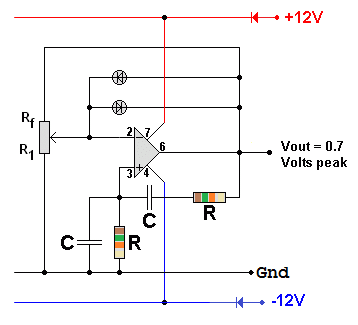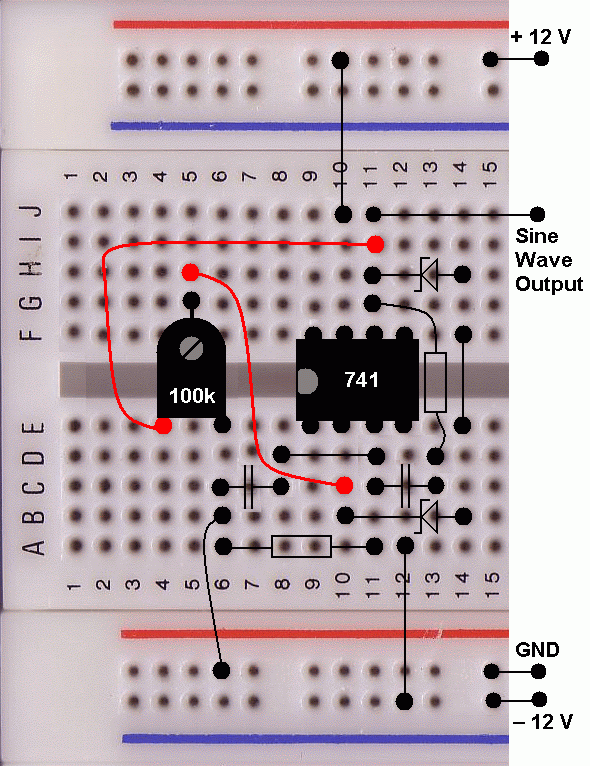RANDOM PAGE

SITE SEARCH

LOG
IN

HELP

# Sine Wave Oscillator

This is the AQA version closing after June 2019. Visit the the version for Eduqas instead.• Rf and R1 = 100 k pre-set potentiometer - The slider is set about a quarter of the way round. This is used to control the gain. The gain should be as small as possible consistent with the oscillator starting up reliably.
• R = 15 k - This controls the oscillator frequency.
• C = 10nF - This also controls the oscillator frequency.
• The Zener diodes limit the oscillator output by decreasing the amplifier gain if the output rises above their breakdown voltage. They also decrease the accuracy of the sine wave shape. However if the diodes are not there, the amplifier limits and the distortion is much worse.
• For a much smaller audio output, replace the Zener diodes in series with normal diodes in parallel. The output will now be about +/- 0.7 volts, suitable for headphones or the input of an audio amplifier.
• This oscillator runs at about 1kHz. Use the formula below ...
• F = 1 / (2 π RC)

This circuit is designed to have a gain of exactly 1. If the gain were less, the oscillations would die away. If the gain were more, the pure sine wave would get bigger until the op amp limited and the wave would become clipped. If the output is too small, the diodes don't conduct. This makes the gain higher. If the output is too large, the diodes to conduct and this negative feedback decreases the gain. When the output is just right, the gain is one.

The resistors and capacitors (R and C) provide positive feedback. At the frequency of oscillation, the positive feedback is in phase and this reinforces the oscillations. At other frequencies, this is not the case so the oscillator frequency is controlled.

Rf provides negative feedback. With too much negative feedback, the oscillator would fail to start. With too little feedback, the output would increase until the oscillator limited.

Here is a sample layout on protoboard.There is an alternativre to using diodes to limit the output. Use a low power lamp for R1 and a fixed resistor for R2. The fixed resistor should have a value slightly higher than the cold lamp. This gives a gain of more than one so the oscillator should start. The lamp will heat up until the resistances are equal. The oscillator will continue to run with an output that heats the lamp just enough. The lamp fillament will be warm but not glowing. Use the lowest lamp power rating available. A power op amp might be needed because the feedback current is likely to be more than a normal op amp can provide. The same technique could be used with a thremistor in the R2 position and a fixed resistor in the R1 position.

reviseOmatic V3     Contacts, ©, Cookies, Data Protection and Disclaimers Hosted at linode.com, London